# Separation Logic

Jump to: navigation, search

CVC4 supports a syntax for separation logic as an extension of the *.smt2 language.

# Signature

Given a (decidable) base theory T, CVC4 has a decision procedure for quantifier-free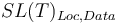$SL(T)_{Loc,Data}$ formulas, where "Loc" and "Data" are any sort belonging to T. A$SL(T)_{Loc,Data}$ formula is one from the following grammar:

 F : L | (emp t u) | (pto t u) | (sep F1 ... Fn) | (wand F1 F2) | ~F1 | F1 op ... op Fn


where "op" is any classical Boolean connective, t and u are terms built from symbols in the signature of T of sort Loc and Data respectively, and L is a T-literal. The operator "emp" denotes the empty heap constraint, the operator "pto" denotes the points-to predicate, the operator "sep" denotes separation start and is variadic, and the operator "wand" denote magic wand.

# Semantics

A satisfiability relation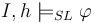$I,h \models_{SL} \varphi$ is defined for$SL(T)_{Loc,Data}$ formulas$\varphi$, where I is an interpretation, and h is a heap. The semantics of separation logic operators are as follows: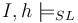$I,h \models_{SL}$ L Iff$I \models$ L, if L is a T-literal$I,h \models_{SL}$ (emp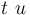$t \ u$) Iff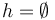$h = \emptyset$$I,h \models_{SL}$ (pto$t \ u$) Iff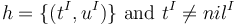$h = \{(t^I,u^I)\} \text{ and } t^I\not=nil^I$$I,h \models_{SL}$ (sep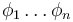$\phi_1 \ldots \phi_n$) Iff there exist heaps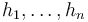$h_1,\ldots,h_n$ s.t.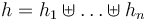$h=h_1\uplus \ldots \uplus h_n$ and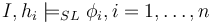$I,h_i \models_{SL} \phi_i, i = 1,\ldots,n$$I,h \models_{SL}$ (wand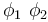$\phi_1 \ \phi_2$) Iff for all heaps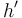$h'$ if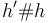$h'\#h$ and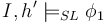$I,h' \models_{SL} \phi_1$ then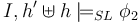$I,h'\uplus h \models_{SL} \phi_2$

where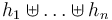$h_1 \uplus \ldots \uplus h_n$ denotes the disjoint union of heaps$h_1, \ldots, h_n$ and$h'\#h$ denotes that heaps h' and h are disjoint, and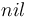$nil$ is a distinguished variable of sort Loc. All classical Boolean connectives are interpreted as expected. Note that the arguments of "emp" are used to denote the type of the heap and have no meaning otherwise.

# Syntax

Separation logic in CVC4 requires the "all supported" logic:

 (set-logic QF_ALL_SUPPORTED)


The syntax for the operators of separation logic is summarized in the following table:

CVC language SMTLIB language C++ API
Empty heap N/A (_ emp T1 T2) em.mkExpr(kind::SEP_EMP, X, Y); where X and Y are of type T1 and T2.
Points-to N/A (pto X Y) em.mkExpr(kind::SEP_PTO, X, Y);
Separation star N/A (sep C1 ... Cn) em.mkExpr(kind::SEP_STAR, C1, ..., Cn);
Magic wand N/A (wand C1 C2) em.mkExpr(kind::SEP_WAND, C1, C2);
Nil element N/A (as nil T) em.mkUniqueVar(T,kind::SEP_NIL);

# Examples

The following input on heaps Int -> Int is unsatisfiable:

 (set-logic QF_ALL_SUPPORTED)
(set-info :status unsat)
(declare-const x Int)
(declare-const a Int)
(declare-const b Int)
(assert (and (pto x a) (pto x b)))
(assert (not (= a b)))
(check-sat)


The following input on heaps U -> Int is satisfiable. Notice that the formula (not (emp x 0)) is satisfied by heaps U -> Int (the types of x and 0 respectively) whose domain is non-empty.

 (set-logic QF_ALL_SUPPORTED)
(set-info :status unsat)
(declare-sort U 0)
(declare-const x U)
(declare-const a Int)
(assert (and (not (_ emp Int U)) (pto x a))
(check-sat)


The following input on heaps Int -> Node is satisfiable, where Node denotes a user-defined inductive datatype:

 (set-logic QF_ALL_SUPPORTED)
(set-info :status sat)
(declare-const x Int)
(declare-const y Int)
(declare-const z Int)
(declare-datatypes () ((Node (node (data Int) (left Int) (right Int)))))
(assert (pto x (node 0 y z)))
(check-sat)


Given a separation logic input, the sorts Loc and Data are inferred by CVC4, and must be consistent across all predicates occurring in an input. CVC4 does not accept an input such as:

 (set-logic QF_ALL_SUPPORTED)
(declare-sort U 0)
(declare-const x U)
(assert (and (pto x 0) (pto 1 2)))


since the sorts of the first arguments of the points-to predicates do not agree.